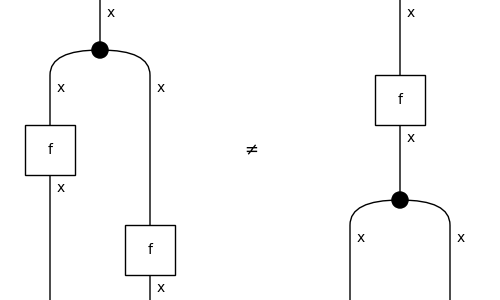# Diagram#

class discopy.markov.Diagram(inside, dom, cod, _scan=True)[source]#

A Markov diagram is a symmetric diagram with `Copy` boxes.

Parameters:
• inside (Layer) – The layers inside the diagram.

• dom (monoidal.Ty) – The domain of the diagram, i.e. its input.

• cod (monoidal.Ty) – The codomain of the diagram, i.e. its output.

Note

We can create arbitrary Markov diagrams with the standard notation for Python functions.

```>>> x = Ty('x')
>>> f = Box('f', x, x)
```
```>>> copy_then_apply = Diagram.from_callable(x, x @ x)(
...     lambda x: (f(x), f(x)))
```
```>>> @Diagram.from_callable(x, x @ x)
... def apply_then_copy(x):
...     y = f(x)
...     return x, x
```
```>>> from discopy.drawing import Equation
>>> Equation(copy_then_apply, apply_then_copy, symbol="\$\\neq\$").draw(
...     path="docs/_static/markov/copy_and_apply.png")
```classmethod copy(x, n=2)[source]#

Make `n` copies of a given type `x`.

Parameters:
• x (Ty) – The type to copy.

• n – The number of copies.

Return type:

Diagram

classmethod merge(x, n=2)[source]#

Merge `n` copies of a given type `x`.

Parameters:
• x (Ty) – The type to copy.

• n – The number of copies.

Return type:

Diagram

The discard of an atomic type `x`.

Parameters:

x (Ty) – The type to discard.

Return type:

Diagram

braid_factory#

alias of `Swap`

copy_factory#

alias of `Copy`

alias of `Discard`

factory#

alias of `Diagram`

merge_factory#

alias of `Merge`

sum_factory#

alias of `Sum`

trace_factory#

alias of `Trace`